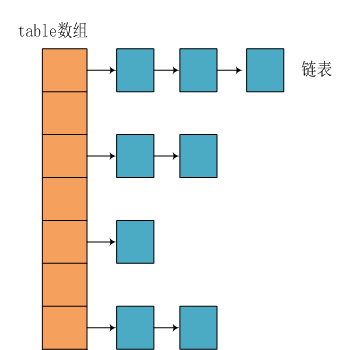# java面试题-Java集合相关java面试题-Java集合相关1. ArrayList 和 Vector 的区别

ArrayList和Vector底层实现原理都是一样得,都是使用数组方式存储数据

Vector是线程安全的，但是性能比ArrayList要低。

ArrayList，Vector主要区别为以下几点：

（1）：Vector是线程安全的，源码中有很多的synchronized可以看出，而ArrayList不是。导致Vector效率无法和ArrayList相比；

（2）：ArrayList和Vector都采用线性连续存储空间，当存储空间不足的时候，ArrayList默认增加为原来的50%，Vector默认增加为原来的一倍；

（3）：Vector可以设置capacityIncrement，而ArrayList不可以，从字面理解就是capacity容量，Increment增加，容量增长的参数。

ArrayList采用的数组形式来保存对象，这种方法将对象放在连续的位置中，所以最大的缺点就是插入和删除的时候比较麻烦，查找比较快；

Vector使用了sychronized方法(线程安全),所以在性能上比ArrayList要差些.

3.快速失败 (fail-fast) 和安全失败 (fail-safe) 的区别是什么？

1.快速失败

```        @SuppressWarnings("unchecked")
public E next() {
checkForComodification();
int i = cursor;
if (i >= size)
throw new NoSuchElementException();
Object[] elementData = ArrayList.this.elementData;
if (i >= elementData.length)
throw new ConcurrentModificationException();
cursor = i + 1;
return (E) elementData[lastRet = i];
}```
```        final void checkForComodification() {
if (modCount != expectedModCount)
throw new ConcurrentModificationException();
}```

2.安全失败

原理：

Java.util.concurrent包下的容器都是安全失败的，可以在多线程下并发使用，并发修改。

4.HashMap 的数据结构

HashMap的主干类是一个Entry数组(jdk1.7) ,每个Entry都包含有一个键值队(key-value).

```static class Entry<K,V> implements Map.Entry<K,V> {
final K key;
V value;
Entry<K,V> next;//存储指向下一个Entry的引用，单链表结构
int hash;//对key的hashcode值进行hash运算后得到的值，存储在Entry，避免重复计算

/**
* Creates new entry.
*/
Entry(int h, K k, V v, Entry<K,V> n) {
value = v;
next = n;
key = k;
hash = h;
}```5.HashMap 的工作原理

HashMap基于hashing原理，我们通过put()和get()方法存储和获取对象，当我们将键值对传递给put()方法时，它调用键对象的hashCode()方法来计算hashcode,让后找到bucket位置来存储值对象。当获取对象时，通过键对象的equals()方法找到正确的键值对，然后返回对象。

```public V put(K key, V value) {
//当key为null，调用putForNullKey方法，保存null与table第一个位置中，这是HashMap允许为null的原因
if (key == null)
return putForNullKey(value);
//计算key的hash值
int hash = hash(key.hashCode());                  //计算key hash 值在 table 数组中的位置
int i = indexFor(hash, table.length);             //从i出开始迭代 e,找到 key 保存的位置
for (Entry<K, V> e = table[i]; e != null; e = e.next) {
Object k;
//判断该条链上是否有hash值相同的(key相同)
//若存在相同，则直接覆盖value，返回旧value
if (e.hash == hash && ((k = e.key) == key || key.equals(k))) {
V oldValue = e.value;    //旧值 = 新值
e.value = value;
e.recordAccess(this);
return oldValue;     //返回旧值
}
}
//修改次数增加1
modCount++;
//将key、value添加至i位置处
return null;
}```

get()源码:

```public V get(Object key) {
// 若为null，调用getForNullKey方法返回相对应的value
if (key == null)
return getForNullKey();
// 根据该 key 的 hashCode 值计算它的 hash 码
int hash = hash(key.hashCode());
// 取出 table 数组中指定索引处的值
for (Entry<K, V> e = table[indexFor(hash, table.length)]; e != null; e = e.next) {
Object k;
//若搜索的key与查找的key相同，则返回相对应的value
if (e.hash == hash && ((k = e.key) == key || key.equals(k)))
return e.value;
}
return null;
}```

6.Hashmap 什么时候进行扩容呢？

这里我们再来复习put的流程：当我们想一个HashMap中添加一对key-value时，系统首先会计算key的hash值，然后根据hash值确认在table中存储的位置。若该位置没有元素，则直接插入。否则迭代该处元素链表并依此比较其key的hash值。如果两个hash值相等且key值相等(e.hash == hash && ((k = e.key) == key || key.equals(k))),则用新的Entry的value覆盖原来节点的value。如果两个hash值相等但key值不等 ，则将该节点插入该链表的链头。具体的实现过程见addEntry方法，如下：

```void addEntry(int hash, K key, V value, int bucketIndex) {
//获取bucketIndex处的Entry
Entry<K, V> e = table[bucketIndex];
//将新创建的 Entry 放入 bucketIndex 索引处，并让新的 Entry 指向原来的 Entry
table[bucketIndex] = new Entry<K, V>(hash, key, value, e);
//若HashMap中元素的个数超过极限了，则容量扩大两倍
if (size++ >= threshold)
resize(2 * table.length);
}```

一是链的产生。这是一个非常优雅的设计。系统总是将新的Entry对象添加到bucketIndex处。如果bucketIndex处已经有了对象，那么新添加的Entry对象将指向原有的Entry对象，形成一条Entry链，但是若bucketIndex处没有Entry对象，也就是e==null,那么新添加的Entry对象指向null，也就不会产生Entry链了。

二、扩容问题。

7.HashSet怎样保证元素不重复

都知道HashSet中不能存放重复的元素,有时候可以用来做去重操作。但是其内部是怎么保证元素不重复的呢？

```public class HashSet<E>
extends AbstractSet<E>
implements Set<E>, Cloneable, java.io.Serializable
{
static final long serialVersionUID = -5024744406713321676L;

private transient HashMap<E,Object> map;

private static final Object PRESENT = new Object();

public HashSet() {
map = new HashMap<>();
}
...
}```

HashSet的构造方法其实就是在内部实例化了一个HashMap对象，其中还会看到一个static final的PRESENT变量；

```    public boolean add(E e) {
return map.put(e, PRESENT)==null;
}```

```public V put(K key, V value) {
if (table == EMPTY_TABLE) {
inflateTable(threshold);
}
if (key == null)
return putForNullKey(value);
int hash = hash(key);
int i = indexFor(hash, table.length);
for (Entry<K,V> e = table[i]; e != null; e = e.next) {
Object k;
if (e.hash == hash && ((k = e.key) == key || key.equals(k))) {
V oldValue = e.value;
e.value = value;
e.recordAccess(this);
return oldValue;
}
}

modCount++;
return null;
}```

`if (e.hash == hash && ((k = e.key) == key || key.equals(k)))`• 回顶